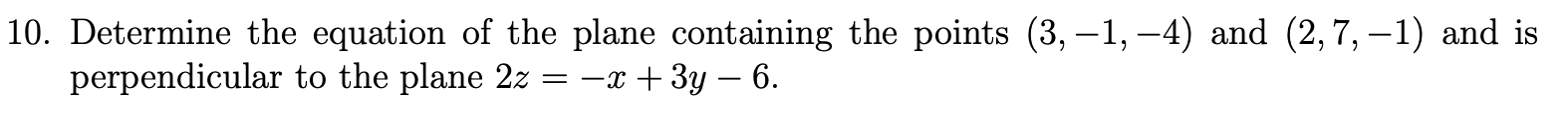Home / Expert Answers / Calculus / 0-determine-the-equation-of-the-plane-containing-the-points-3-1-4-and-2-7-1-and-is-pa805

# (Solved): 0 . Determine the equation of the plane containing the points (3,1,4) and (2,7,1) and is ...0 . Determine the equation of the plane containing the points and and is perpendicular to the plane .

We have an Answer from Expert